MORE IN Basic Electrical & Electronics Engg
MU First Year Engineering (Semester 1)
Basic Electrical & Electronics Engg
May 2013
Total marks: --
Total time: --
INSTRUCTIONS
(1) Assume appropriate data and state your reasons
(2) Marks are given to the right of every question
(3) Draw neat diagrams wherever necessary

1(a) Using source transformation convert the circuit given below to a single voltage source in series with a resistor.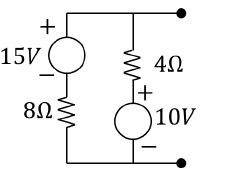3 M
1(b) Derive the condition for maximum power transfer through the network.
3 M
1(c) Determine the rms value of voltage waveform shown below:-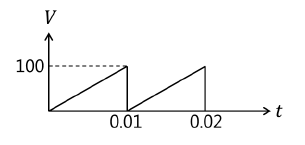3 M
1(d) Give the comparison between series and parallel resonance circuits.
3 M
1(e) Draw a phasor diagram with 3-phase star connected load with lagging power factor.
2 M
1(f) State the working principle of transformer and derive the expression for emf induced.
4 M
1(g) Define Ripple Factor and Voltage regulation for rectifier circuits.
2 M

2(a) For the network given below find the current through the 3Ω resistor using nodal analysis.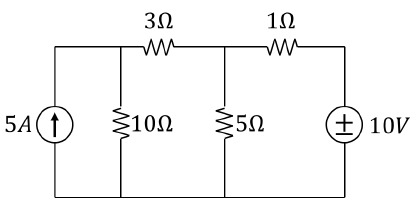6 M
2(b) Two coils A and B are connected in series across 240V, 50Hz supply. The resistance of A is 5Ω and the inductance of B is 0.015H. If the input from supply is 3kW and 2 kVAR, find the inductance of A and resistance of B. Calculate the voltage across each coil.
8 M
2(c) A 3000/200 V, 50 Hz single phase transformer has a cross sectional area of 150 cm2 for the core. If the number of turns on the low voltage winding is 80, determine number of turns on the high voltage winding and maximum value of flux density in the core.
6 M

3(a) Each phase of a delta connected load consist of a 50mH inductor in series with a parallel combination of 5Ω resistor and a 5μF capacitor. The load is is connected to a three phase, 550 V, 50Hz ac supply.
Find:-
(i) Phase current (ii) Line current
(iii) Power drawn (iv) power factor
(v) reactive power and kVA rating of the load.
8 M
3(b) A 5kVA, 1000/200 V, 50 Hz, single phase transformer gives following test results:
 OC Test (LV Side) 200V 1.2A 90W SC Test (HV Side) 50V 5A 110W
Determine efficiency as half load at 0.8 p.f. lagging.
6 M
3(c) What is the function of filter in rectifier circuits. Explain with appropriate waveforms.
2 M
3(d) Draw and explain Output characteristics of transistor in CE configuration.
4 M

4(a) For the circuit shown below find the resistance between terminals A and B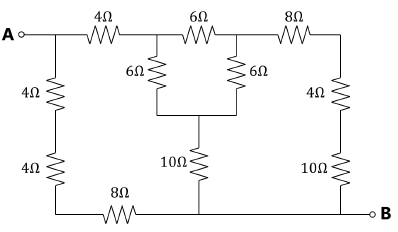7 M
4(b) The voltage drops across four series connected impedances are given
V1=60 sin(ωt + π/6);
V2=75 sin(ωt - 5π/6);
V3=100 cos(ωt + π/4);
V4=V4m sin(ωt+ ϕ4).
Calculate the values of V4m and ϕ4 if the voltage applied across series circuit is
140 sin(ωt + 3π/5).
5 M
4(c) Draw the circuit for measurement of 3 phase power using two wattmeters and state the advantages over other methods of 3-phase power measurement.
4 M
4(d) Draw and explain Half wave rectifier with appropriate waveforms.
4 M

5(a) Using Norton's theorem, calculate the current flowing through 15 Ω load resistor in the given circuit.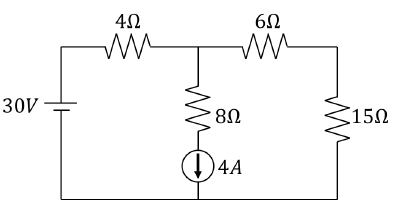8 M
5(b) A 46mH inductive coil has a resistance of 10 Ω.
(i) How much current will it draw if connected across a 100V,60Hz supply?
(ii) What is the power factor of the coil?
(iii) Determine the value of capacitance that must be connected across the coil to make the power factor of overall circuit unity.
4 M
5(c) A 30 kVA, 2400/120V, 50Hz, transformer has a high voltage winding resistance of 9.1Ω and a leakage reactance of 0.22Ω. The low voltage winding resistance is 0.035Ω and the leakage reactance is 0.012Ω. Calculate for the transformer:
(i) Equivalent resistance as referred to both primary and secondary
(ii) Equivalent reactance as referred to both primary and secondary
(iii) Equivalent impedance as referred to both primary and secondary
(iv) Copper loss at full load.
8 M

6(a) Determine current through RL = 2Ω in the circuit shown below using superposition theorem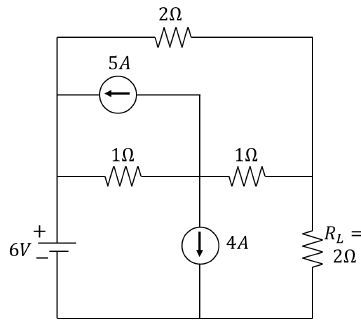7 M
6(b) An inductor having a resistance of 25Ω and Q0 of 10 at resonant frequency of 10 kHz is fed from 100 ?00 supply. Calculate
(i) Value of series capacitance required to produce resonance with the coil.
(ii) The inductance of the coil.
(ii) Q0 using L/C Ratio.
(iv) Voltage across capacitor.
(v) Voltage across coil.
7 M
6(c) The input power of 3-phase motor was measured by two wattmeter method. The reading of two wattmeters are 5.2kW and -1.7kW and the line voltage is 415V. Calculate the total Active Power, Power factor and line current.
6 M

More question papers from Basic Electrical & Electronics Engg